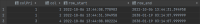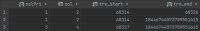# ROW START not always set correctly in transaction-precise tables

XMLWordPrintable

#### Details

•Bug
• Status: Open
•Major
• Resolution: Unresolved
• 10.9.3
• None
• None
• Docker

#### Description

For transaction-precise system-versioned tables, when a user makes a "non-update" (i.e. update a value in a row to the same value), the ROW START ID is not set to the current transaction for the updated row.

Here are two system verisioned tables, one "normal" and one transaction-precise:

 `DROP TABLE IF EXISTS timebased;` `CREATE TABLE timebased (colPri INT NOT NULL PRIMARY KEY, col int) WITH SYSTEM VERSIONING;` `INSERT INTO timebased VALUES (1, 2);` `INSERT INTO timebased VALUES (3,4);` ` ` `DROP TABLE IF EXISTS transactionbased;` `CREATE TABLE transactionbased (colPri INT NOT NULL PRIMARY KEY, col int, trx_start BIGINT UNSIGNED GENERATED ALWAYS AS ROW START,` ` trx_end BIGINT UNSIGNED GENERATED ALWAYS AS ROW END,` ` PERIOD FOR SYSTEM_TIME(trx_start, trx_end)) WITH SYSTEM VERSIONING;` `INSERT INTO transactionbased (colPri, col) VALUES (1, 2);` ```INSERT INTO transactionbased (colPri, col) VALUES (3,4); ```

We then update the column "col" to the same value for one of the rows and get the resulting table with history. First for the timebased table:

 `UPDATE timebased SET col = 2 WHERE colPri = 1;` ```SELECT colPri, col, row_start, row_end FROM timebased FOR SYSTEM_TIME ALL; ```

This gives the expected result:The updated row shows a "row_start" as the old one ends, and the max-value for "row_end"

But if we do the same for the transactionbased table:

 `UPDATE transactionbased SET col = 2 WHERE colPri = 1;` ```SELECT * FROM transactionbased FOR SYSTEM_TIME ALL; ```

The result is quite different:trx_start for the updated row is exactly the same as for the original row.

Is this on purpose? It seems quite... Wrong. Now, in normal "what was it at that time", I don't think it matters much, and I haven't seen issues around it... But when you're trying to get a list of changes (at least the way I do it), it is very annoying. My only workaround is this WHERE clause to get the changed rows for a specific transaction (:trxid is the value that I'm looking for)

 `WHERE :trxid = trx_start OR (:trxid = trx_end AND` ``` ID NOT IN (SELECT ID FROM someTable FOR SYSTEM_TIME AS OF TRANSACTION :trxid t2 WHERE t2.trx_start <> someTable.trx_end)); ```

If I simply used

 `WHERE :trxid = start_trxid OR :trxid = end_trxid`

(as I could without the issue), it would look like the row was deleted, as I'd only get the old row and not the new one.

#### PeopleUnassignedDennis DK